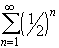#Interactive Real Analysis

Next | Previous | Glossary | Map

## 4.1. Series and Convergence

### Examples 4.1.3(a):

The infinite series= 1/2 + 1/4 + 1/8 + 1/16 + ... converges to 1 (this series is a special case of the geometric series).
The n-th partial sum for this series is defined as
S n = 1/2 + 1/2 2 + 1/2 3 + ... + 1/2 n

### You browser is not 'Java-enabled' ...

We need to find a closed form for this expression to be able to take the limit of the sequence of partial sums.

If we divide the above expression by 2 and then subtract it from the original one we get:

S n - 1/2 S n = 1/2 - 1/2 n+1
Hence, solving this for S n we obtain
S n = 2 (1/2 - 1/2 n+1)
This is now a sequence, and we can take the limit as n goes to infinity. By our result on the power sequence, the term 1/2 n+1 goes to zero, so that
lim S n = 1
That proves, by definition, that the infinite series converges to the number 1.Next | Previous | Glossary | Map